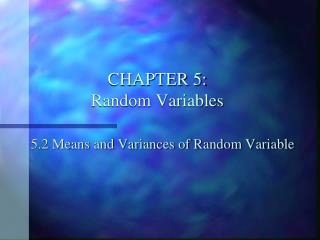# CHAPTER 5: Random Variables - PowerPoint PPT PresentationDownload PresentationCHAPTER 5: Random Variables

CHAPTER 5: Random VariablesDownload Presentation## CHAPTER 5: Random Variables

- - - - - - - - - - - - - - - - - - - - - - - - - - - E N D - - - - - - - - - - - - - - - - - - - - - - - - - - -
##### Presentation Transcript

1. CHAPTER 5:Random Variables 5.2 Means and Variances of Random Variable

2. PICK 3 • You buy a Pick 3 ticket and choose straight play. • How much does it cost? • How much could you win? • What is the probability of winning with that one ticket? • What is the average payoff?

3. Means • Mean of a DISCRETE Random Variable. • This is sometimes called the expected value. • In the lottery problem, is it possible to win or lose just \$.50? • The expected value is often not a possible value of X.

4. Find the mean of the number of dots that appear when a die is tossed. X P(X) X * P(X)

5. In a family with three children, find the mean number of children who will be girls. • X P(X)

6. Class work/ Homework • P. 267 numbers 3, 4, and 5 • ONLY FIND THE MEAN!

7. Variance • Variance of a DISCRETE Random Variable. • The standard deviation is the square root of the variance.

8. Variance Find the variance and standard deviation for the number of spots that appear when a die is tossed. (Recall from yesterday that the mean is 3.5) X P(X) X* P(X) X2 * P(X)

9. Variance • Take a look at problem 2 on page 267…

10. Means and Variancesof Random Variables • page 267 #1, 6-10

11. Expected Value • The expected value of a discrete random variable of a probability distribution is the theoretical average of the variable • E(X) = expected value

12. Example • One thousand tickets are sold at \$1 each for a color TV valued at \$350. What is the expected value of the gain if you purchase one ticket?

13. Example 2 A client has two bonds to choose from in which to invest \$5000. Bond X pays a return of 4% and has a default rate of 2%. Bond Y pays a 2 ½ % return and has a default of 1%. Find the expected rate of return and decide which bond would be a better investment if the investor loses all the investment when the bond defaults.

14. Practice • P. 268 14-18